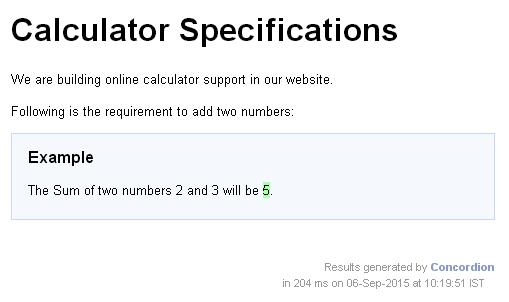# Concordion - execute Command

Concordion execute command is used run the operation of concordion fixture. Consider the following requirement −
```The sum of two numbers 2 and 3 will be 5.
```
If we want to write a specification for a sum function which will accept two numbers and output their sum, then the specification will be as follows −
```<p>The Sum of two numbers <span concordion:set = "#firstNumber">2</span> and
<span concordion:set = "#secondNumber">3</span> will be
<span concordion:execute = "#result = sum(#firstNumber, #secondNumber)">
</span><span concordion:assertEquals = "#result">5</span>.</p>```
When Concordion parses the document, it will set a temporary variable #firstNumber to be the value "2" and #secondNumber to be the value "3" and then execute the sum() method with parameters as #firstNumber and #secondNumber using the execute command and set the result into #result variable and check that the #result variable is equal to "5".

### Example

Let us have a working Eclipse IDE in place and follow the steps given below to create a Concordion application −
StepDescription
1Create a project with a name concordion and create a package com.tutorialspoint under the src folder in the created project.
2Add required Concordion libraries using Add External JARs option as explained in the Concordion - First Application chapter.
3Create Java class System under the com.tutorialspoint package.
4Create Fixture class SystemFixture under the specs.tutorialspoint package.
5Create Specification html System.html under the specs.tutorialspointpackage.
6The final step is to create the content of all the Java files and specification file and run the application as explained below.
Here is the content of the System.java file −
```package com.tutorialspoint;
public class System {
public int sum(int firstNumber, int secondNumber) {
return firstNumber + secondNumber;
}
}```
Following is the content of the SystemFixture.java file −
```package specs.tutorialspoint;

import org.concordion.integration.junit4.ConcordionRunner;
import org.junit.runner.RunWith;
import com.tutorialspoint.System;

@RunWith(ConcordionRunner.class)

public class SystemFixture {
System system = new System();
public int sum(int firstNumber, int secondNumber) {
return system.sum(firstNumber, secondNumber);
}
}```
Following is the content of the System.html file −
```<html xmlns:concordion = "http://www.concordion.org/2007/concordion">
<link href = "../concordion.css" rel = "stylesheet" type = "text/css" />

<body>
<h1>Calculator Specifications</h1>
<p>We are building online calculator support in our website.</p>
<p>Following is the requirement to add two numbers:</p>

<div class = "example">
<h3>Example</h3>
<p>The Sum of two numbers <span concordion:set = "#firstNumber">2</span>
and <span concordion:set = "#secondNumber">3</span> will be
<span concordion:execute = "#result = sum(#firstNumber, #secondNumber)">
</span><span concordion:assertEquals = "#result">5</span>.</p>
</div>

</body>

</html>```
Once you are done with creating source and specification files, let us run the application as JUnit Test. If everything is fine with your application, then it will produce the following result −
```C:\DOCUME~1\ADMINI~1\LOCALS~1\Temp\concordion\specs\tutorialspoint\System.html
Successes: 1, Failures: 0
```
System.html is the output of Concordion test run.